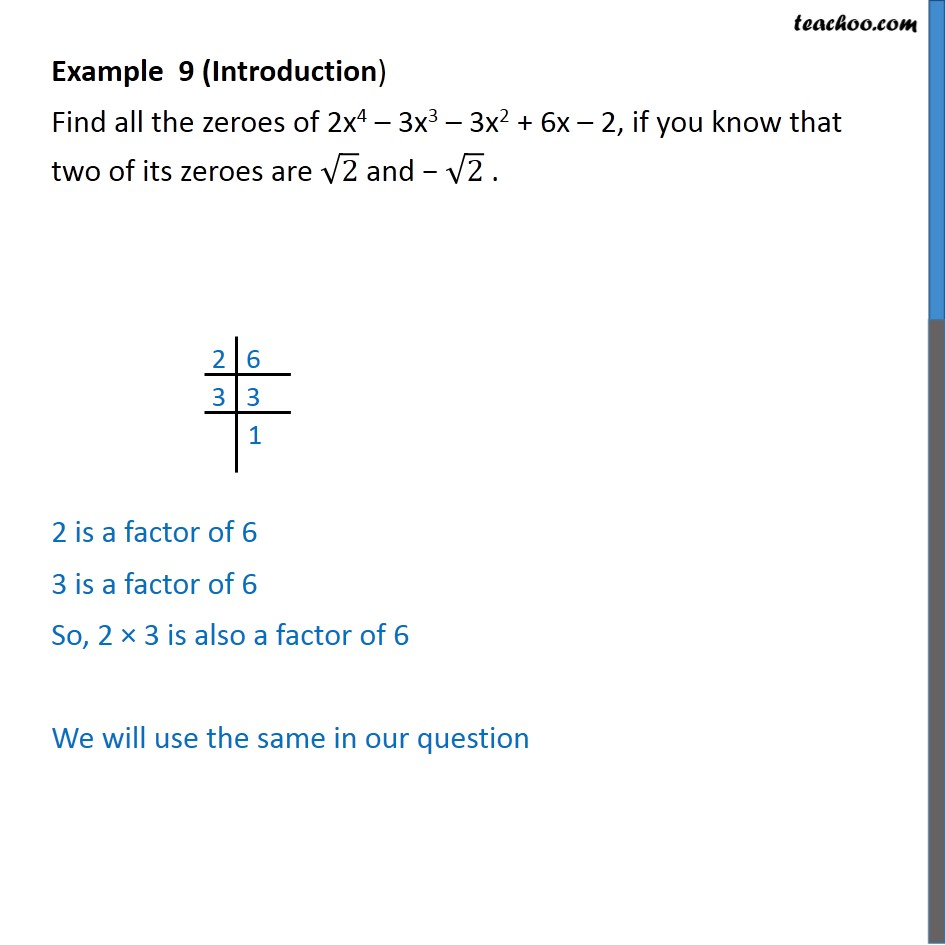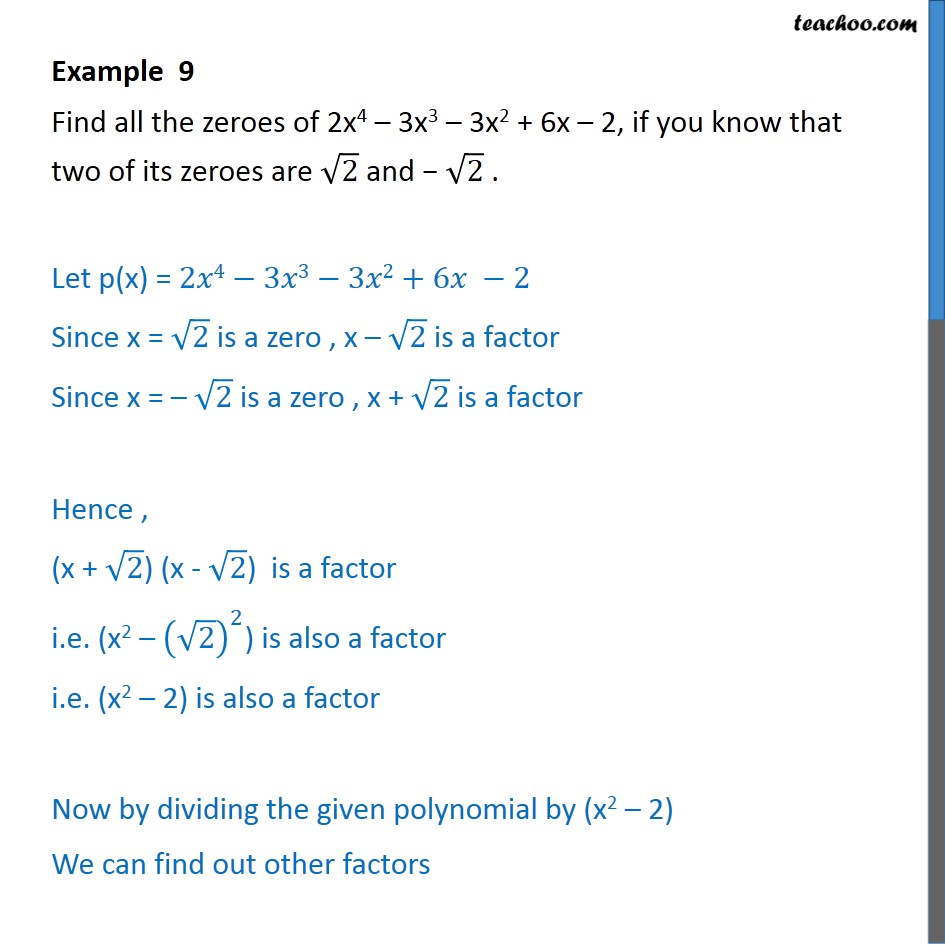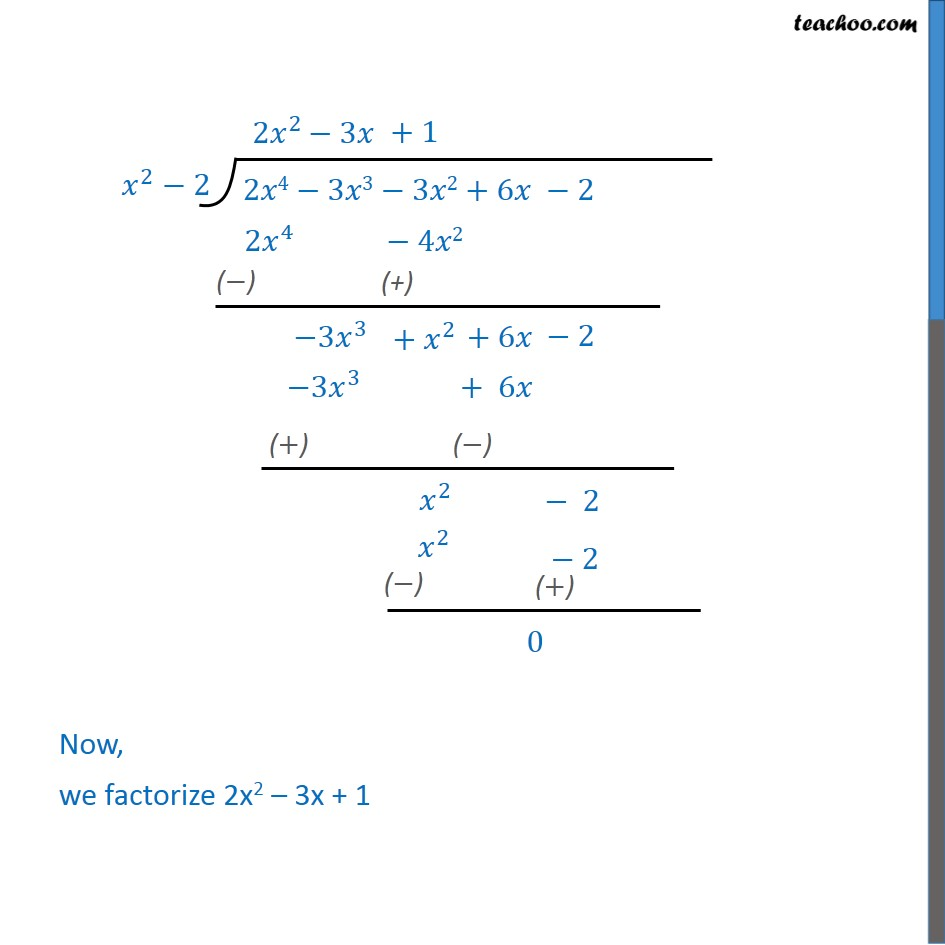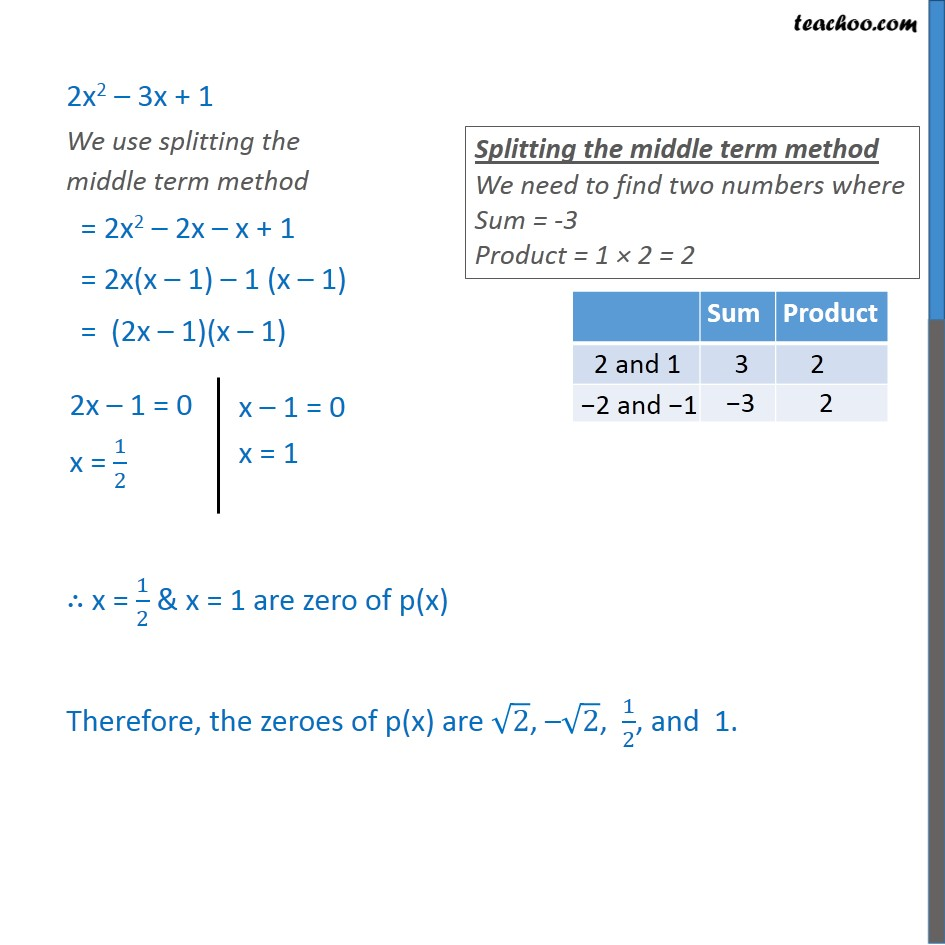Chapter 2 Class 10 Polynomials

Class 10
Important Questions for Exam - Class 10Learn in your speed, with individual attention - Teachoo Maths 1-on-1 Class

### Transcript

Example 9 (Introduction) Find all the zeroes of 2x4 – 3x3 – 3x2 + 6x – 2, if you know that two of its zeroes are √2 and − √2 . 2 is a factor of 6 3 is a factor of 6 So, 2 × 3 is also a factor of 6 We will use the same in our question Example 9 Find all the zeroes of 2x4 – 3x3 – 3x2 + 6x – 2, if you know that two of its zeroes are √2 and − √2 . Let p(x) = 2𝑥4−3𝑥3−3𝑥2+6𝑥 −2 Since x = √2 is a zero , x – √2 is a factor Since x = – √2 is a zero , x + √2 is a factor Hence , (x + √2) (x - √2) is a factor i.e. (x2 – (√2)^2) is also a factor i.e. (x2 – 2) is also a factor Now by dividing the given polynomial by (x2 – 2) We can find out other factors Now, we factorize 2x2 – 3x + 1 2x2 – 3x + 1 We use splitting the middle term method = 2x2 – 2x – x + 1 = 2x(x – 1) – 1 (x – 1) = (2x – 1)(x – 1) ∴ x = 1/2 & x = 1 are zero of p(x) Therefore, the zeroes of p(x) are √2, –√2, 1/2, and 1.